# C++ Program for Insertion Sort

In this article, you will learn and get code to sort an array using the insertion sort technique in C++. The following programs are listed in insertion order:

Before going through these programs, if you're not aware of the steps and logic used behind insertion sort, then refer to the insertion sort algorithm and example to get all the things you need.

## Insertion Sort in C++

To sort an array using the insertion sort technique in C++ programming, you have to ask the user to enter the size of the array and then enter elements of that size in random order. After receiving the inputs, sort the given array in ascending order using insertion sort as shown in the program given below:

The question is, "Write a program in C++ to implement insertion sort." Here is the answer:

```#include<iostream>
using namespace std;
int main()
{
int arr, tot, i, j, k, elem, index;
cout<<"Enter the Size for Array: ";
cin>>tot;
cout<<"Enter "<<tot<<" Array Elements: ";
for(i=0; i<tot; i++)
cin>>arr[i];
for(i=1; i<tot; i++)
{
elem = arr[i];
if(elem<arr[i-1])
{
for(j=0; j<=i; j++)
{
if(elem<arr[j])
{
index = j;
for(k=i; k>j; k--)
arr[k] = arr[k-1];
break;
}
}
}
else
continue;
arr[index] = elem;
}
cout<<"\nThe New Array (Sorted Array):\n";
for(i=0; i<tot; i++)
cout<<arr[i]<<"  ";
cout<<endl;
return 0;
}```

This program was built and runs under the Code::Blocks IDE. Here is its sample run: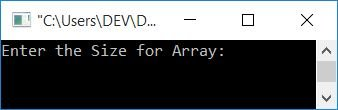Now supply the size, say 10, for the array, and press ENTER. Here is the output you will see: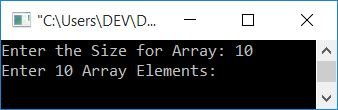Now supply any 10 numbers as 10 array elements, say 10, 1, 9, 2, 8, 3, 7, 4, 6, and 5, one by one. After supplying all 10 inputs, press the ENTER key to sort and print the new array as shown in the output given below: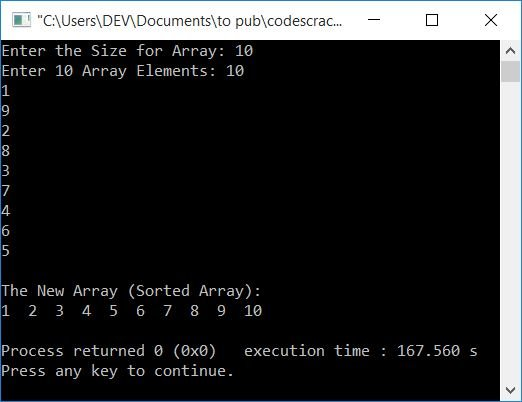The following is how the above program's dry run (receiving user input) goes:

• When the user enters the size, say 10, for the array, it gets stored in tot. So tot=10
• Now we've created a for loop to receive 10 inputs as 10 elements.
• That is, until the condition i<tot (of the for loop) evaluates to false, the statement inside the loop continues to execute.
• And each time we enter the loop, we get a number in the arr.
• At the first run of the for loop, its initialization (first) part executes at first, but only once. As a result, i=0 at first.
• And the condition i<tot gets evaluated. That is, the condition i<tot or 0<10 evaluates to be true, so the program flow goes inside the loop and receives a number from the user. The number gets stored in arr[i] or arr.
• The value of i is now increased. So i=1. And the condition i<tot is evaluated once more with a new value of i.
• Also, the condition i<tot or 1<10 evaluates to true the second time, so another value is received and initialized to arr. In this way, 10 elements gets initialized to arr[] in such a way that the first element gets stored in arr, the second in arr, and so on, up to the tenth element in arr.

And the dry run of insertion sort code in the above program with user input, 10 as size, and 10, 1, 9, 2, 8, 3, 7, 4, 6, 5 as elements of the array, goes like this:

• The for loop starts with 1. As a result, i = 1 at first.
• The condition i<tot or 1<10 evaluates to be true, therefore program flow goes inside the loop, and arr[i] or arr or 1 gets initialized to elem
• Now the condition (of if), elem<arr[i-1] or 1<arr[1-1] or 1<arr or 1<10 evaluates to be true, therefore program flow goes inside if's body.
• There is another for loop; initially j=0 and the condition j<=i or 0<=1 evaluates to be true, therefore program flow goes inside the loop.
• And the condition (of if), elem<arr[j] or 1<arr or 1<10 evaluates to true, program flow enters the if's body, and j or 0 is initialized to index.
• There is one more for loop; with this loop, the value of i (1) gets initialized to k. So k=1, and the condition k>j or 1>=0 evaluates to be true, therefore program flow goes inside the loop, and arr[k-1] or arr[1-1] or arr or 10 gets initialized to arr[k] or arr.
• Now the value of k gets decremented. So k=0
• The condition, k>j or 0>0 evaluates to be false; therefore, execution of this loop gets ended for now. The execution of the outer for loop is skipped when the break keyword is used.
• Now the value of elem (1) gets initialized to arr[index] or arr. So arr=1
• Finally, the two elements, that is, element at the first and second positions, get exchanged. As a result, arr = 1 and arr = 10.
• This process continues, until the condition of the for (the outermost) evaluates to be false.
• Now print the value of arr[], which will be the same array in sorted form.

### Print the array after each sort

After each insertion sort sort, a new array is printed in this program. After watching the output of this program, you can understand its in-depth detail in sorting the elements.

```#include<iostream>
using namespace std;
int main()
{
int arr, tot, i, j, k, elem, index;
cout<<"Enter the Size for Array: ";
cin>>tot;
cout<<"Enter "<<tot<<" Array Elements: ";
for(i=0; i<tot; i++)
cin>>arr[i];
for(i=1; i<tot; i++)
{
elem = arr[i];
if(elem<arr[i-1])
{
for(j=0; j<=i; j++)
{
if(elem<arr[j])
{
index = j;
for(k=i; k>j; k--)
arr[k] = arr[k-1];
break;
}
}
}
else
continue;
arr[index] = elem;
cout<<"\nStep "<<i<<": ";
for(j=0; j<tot; j++)
cout<<arr[j]<<"  ";
}
cout<<"\n\nThe New Array (Sorted Array):\n";
for(i=0; i<tot; i++)
cout<<arr[i]<<"  ";
cout<<endl;
return 0;
}```

Here is its sample run with the same user input as provided in the previous program's sample run: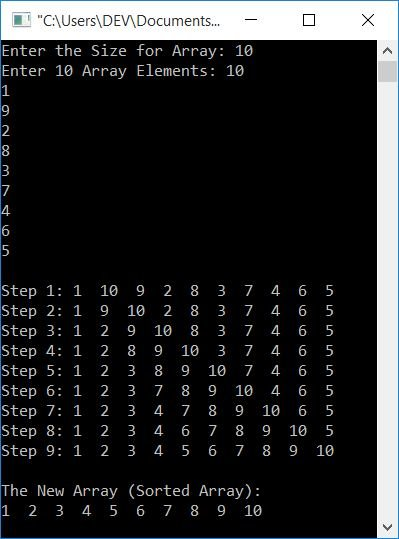## Insertion Sorting with a Function

This is the last program in this article that does the same job as the very first program in this article. The only difference is that this program uses a user-defined function, insertionSort(), to sort an array entered by the user.

This function receives an array and its size as its two arguments.

```#include<iostream>
using namespace std;
void insertionSort(int [], int);
int main()
{
int arr, tot, i;
cout<<"Enter the Size for Array: ";
cin>>tot;
cout<<"Enter "<<tot<<" Array Elements: ";
for(i=0; i<tot; i++)
cin>>arr[i];
insertionSort(arr, tot);
cout<<"\nThe New Array (Sorted Array):\n";
for(i=0; i<tot; i++)
cout<<arr[i]<<"  ";
cout<<endl;
return 0;
}
void insertionSort(int arr[], int tot)
{
int i, elem, j;
for(i=1; i<tot; i++)
{
elem = arr[i];
j = i-1;
while((elem<arr[j]) && (j>=0))
{
arr[j+1] = arr[j];
j--;
}
arr[j+1] = elem;
}
}```

Here is its sample run with user input, 10 as size, and 10, 9, 8, 7, 6, 5, 4, 3, 2, 1 as elements for the array: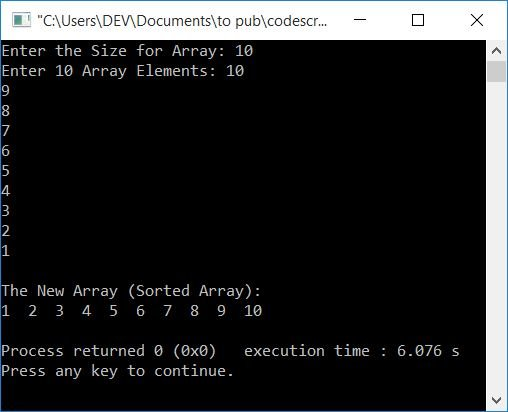#### The same program in different languages

C++ Quiz

« Previous Program Next Program »